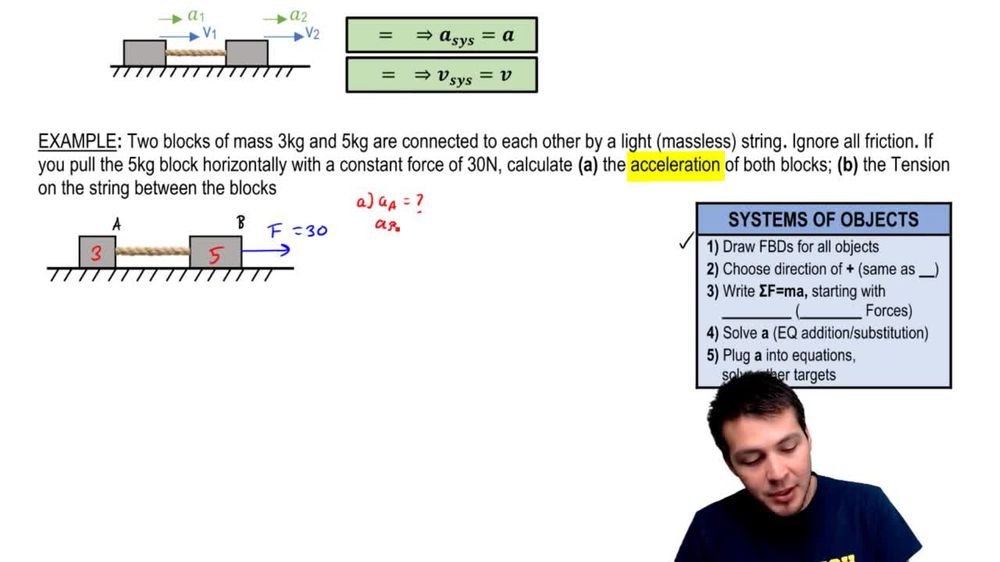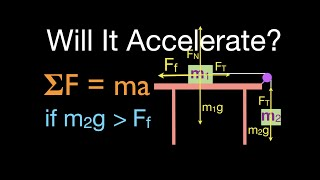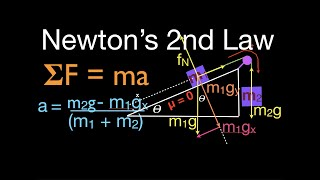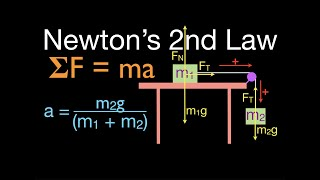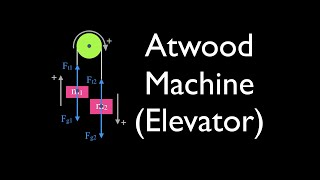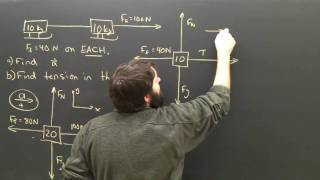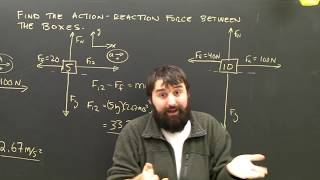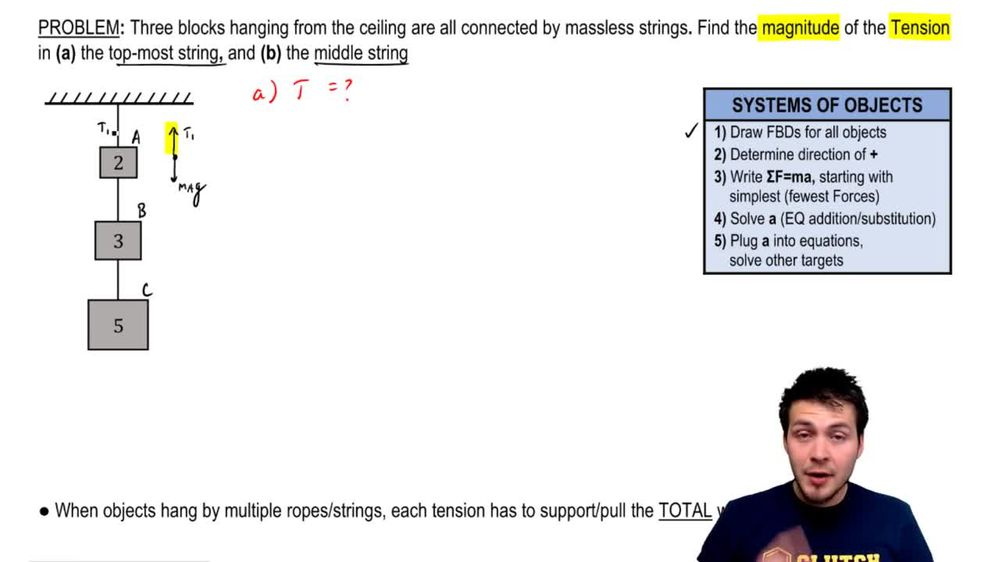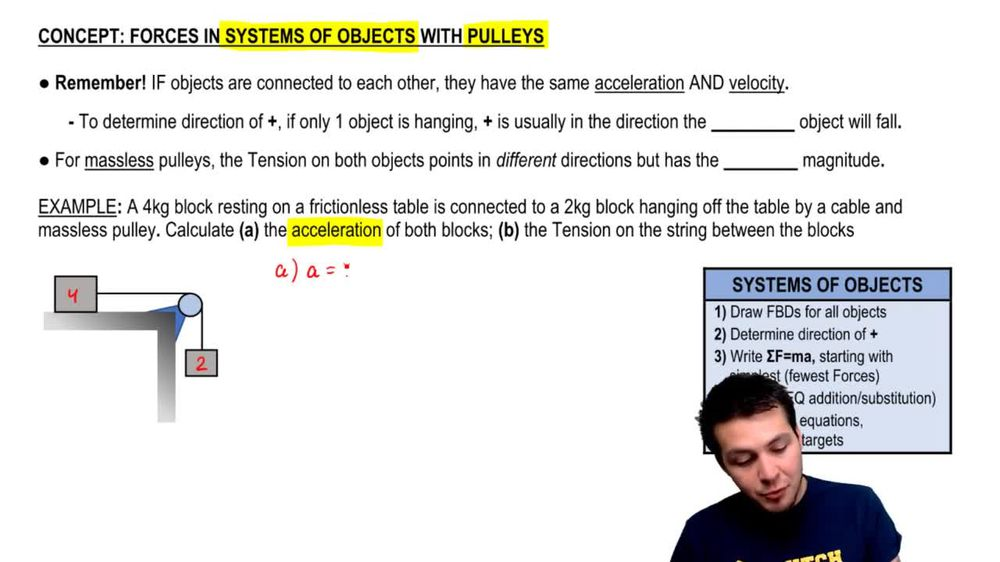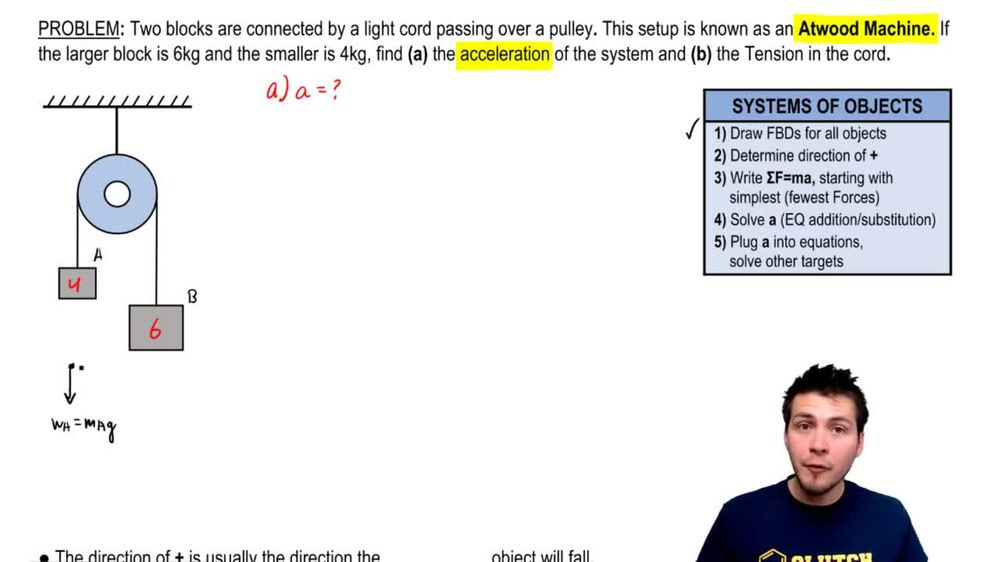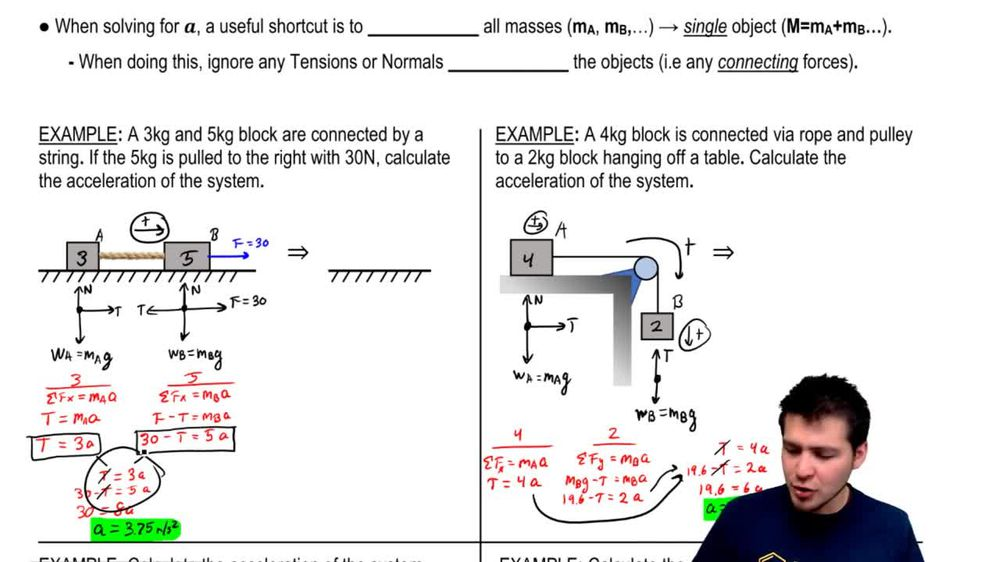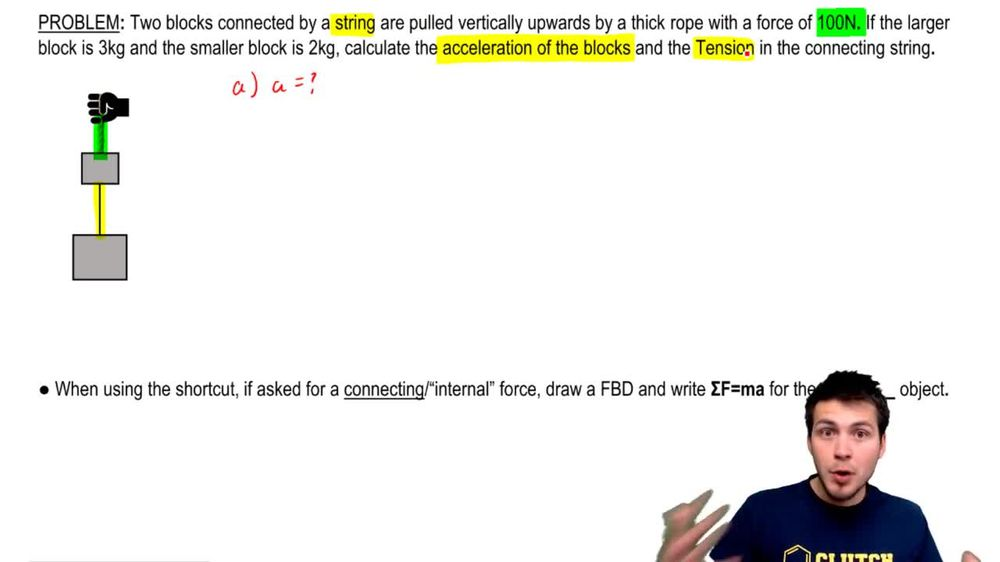Start typing, then use the up and down arrows to select an option from the list.
1. 6. Intro to Forces (Dynamics)2. Forces in Connected Systems of Objects# Pulling Two Connected Blocks Upward

by Patrick Ford
117 views
1
1
Hey, guys, we got these two blocks and they're connected by a string like this. But this whole system here is actually pulled upwards by a rope like this. And you know, this forces 100 Newtons. So we've got the masses of both blocks, but we want to do is we want to calculate the acceleration of the blocks in the first part. So this is really going to be a And in the second part, we're gonna calculate some tension. So we want to calculate the acceleration. We know you can use the shortcut here, So I've got this 2 kg block, this three. So I'm gonna call this one A and B, and what I want to do is just really, really quickly draw a free body diagrams for both of these. So I've got the MSG. That's the weight force that I've got the T rope. That's the tension force from the rope. And I actually know that's 100. And what I've also got is this tension force in the connecting string, and I'll call this T s now for the bottom for the bottom object. I've got the Weight Force MBG and then I got the upward force of the tension and connecting string so we can use the shortcut to find the acceleration. All we're doing really is we're just replacing this block with these two blocks with 15 kg block, right? By adding the masses together, and then we're just gonna ignore any of the internal or the connecting forces. Like the tension in the string. This one doesn't get ignored because it's not actually between the objects. Remember that, right? So basically what happens is is that this one block here is being pulled upwards by t rope, which is 100. And then these weight forces, MSG and MBG can actually combine, right? They don't cancel. And so they combined to actually produce a total weight, which is really just big m times G. So to figure out the acceleration, all we have to do is just use our F equals m a. So then, actually we're going to use f equals big, sometimes a We're going to use the same rules, you know, upwards positive. So any forces along and against get positives and negatives. So our T rope is positive and then minus are big MGs negative and this equals big M A. So this is gonna be 100 times five times 9.8 equals five A. So we ended up getting just 51 equals five A and so a equals 10.2 m per second squared. That's your answer. So you know, this system here is going to accelerate upwards because we got a positive number at 10.2. That's as easy as that, right? You can just go ahead and all these things together in a single object to solve for the acceleration. So let's move on to part B. Now. Now what we want to do is we want to find the tension in this connecting string over here. So this is actually gonna be T s. So here's the deal. Whenever you when you whatever you're solving for the acceleration in these, uh, these kinds of problems, remember, you can always use the shortcut, but if you have to go back and you have to solve for a connecting or internal force, what you're gonna have to do is you're gonna have to draw a free body diagram and write f equals M A. For the simplest object So, for example, here we've got both of these objects that involve t s right. They both have t s in them. So I'm just gonna pick the simplest one, which is really our 3 kg block. So if I write for the 3 kg block, I write f equals M A. Remember, I have to use f equals M A for the little object, right? So I have to use the little M so this is going to be m B times A. So our forces are gonna be t s minus MBG equals M B A. So when you move this to the other side, the M B is actually a common factor, so you can kind of be pulled out of the parentheses, so you've got M. B and this is going to be a plus g. And now you can solve this, right? This is gonna be the massive B, which is three times a, which is 1 10.2 plus 9.8. That's G. And so if you work this now, we're going to get his attention is exactly equal to 60 Newtons. Alright, so it's as simple as that. That's the answer. Let me know if you guys have any questions